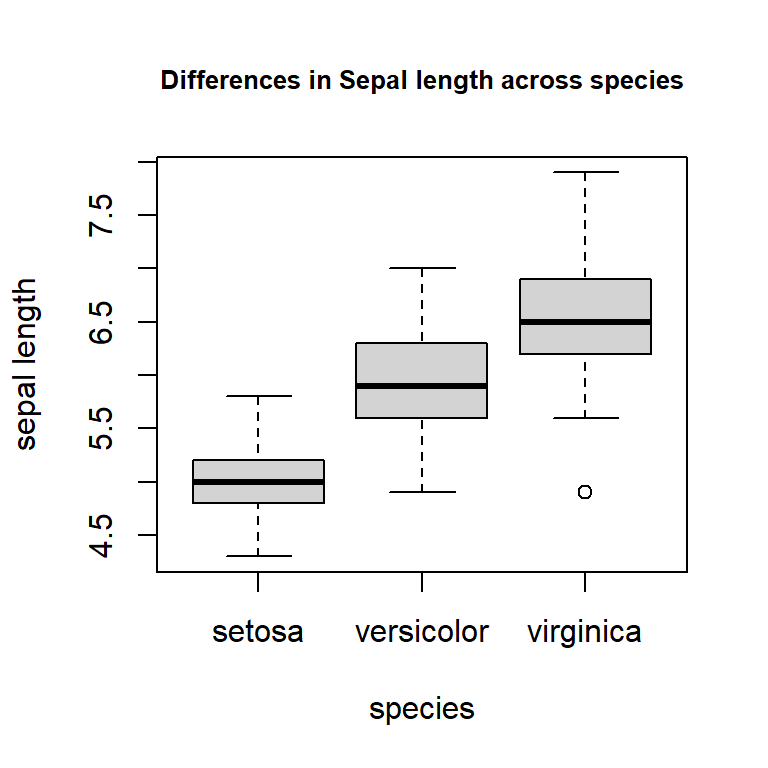### ANOVA with SUMMARY STATISTICS:

Extracted from this document.

As an illustration of the one-way ANOVA calculated from summary statistics in R, we can look at the differences in sepal length between three species of Iris, comparing the output of the “manual” code with the built-in output for the ANOVA table.

We are looking at these differences:

plot(iris$Sepal.Length ~ iris$Species, xlab="species", ylab="sepal length",
main = "Differences in Sepal length across species", cex.main=.8)The SUMMARY STATISTICS (including the number of specimens) are as follows:

    # Number of specimens per species:
(n           = tapply(iris$Sepal.Length, iris$Species, length))
#    setosa versicolor  virginica
# E        50         50         50
# Mean sepal length for each species:
(group_means = tapply(iris$Sepal.Length, iris$Species, mean))
#    setosa versicolor  virginica
#     5.006      5.936      6.588
# Sample variance of the sepal length for each species:
(group_var   = tapply(iris$Sepal.Length, iris$Species, var))
#    setosa versicolor  virginica
#    0.1242490  0.2664327  0.4043429 

Now, working with these summary statistics we can calculate…

The variability between groups:

    # First we need to calculate the overall mean:
grand_mean  = sum(n * group_means) / sum(n)
#... to calculate the sum of square differences between groups:
(SS_between  = sum(n * (group_means - grand_mean)^2))
#  63.21213
# with the corresponding degrees of freedom:
(df_between  = length(unique(iris$Species)) - 1) #  2 # and the mean square error: (MS_between = SS_between / df_between) #  31.60607 Now we can calculate the variability within groups: It’s worth at this point remembering that the sample variance is calculated as $$s^2=\frac{\sum (x_i - \bar x )^2}{n-1}$$ so that the $$\displaystyle \text{SS}_{\text{within groups}}= \sum_{\text{groups}}(n-1) s^2.$$  # We obtained the sum of squares within adding the numerators of the var's): (SS_within = sum((n - 1) * group_var)) #  38.9562 # And calculate the degrees of freedom: (df_within = sum(n - 1)) #  147 # And the mean squared error: (MS_within = SS_within / df_within) #  0.2650082 We calculate the F value and the p value as:  # The ratio of mean squared errors: (F_value = MS_between / MS_within) #  119.2645 # and the corresponding probability: (p_value = pf(F_value, df_between, df_within, lower.tail = F)) #  1.669669e-31 Now we can compare these values to the output in R using the original dataset:  > anova(lm(iris$Sepal.Length ~ iris$Species)) Analysis of Variance Table Response: iris$Sepal.Length
Df Sum Sq Mean Sq F value    Pr(>F)
iris\$Species   2 63.212  31.606  119.26 < 2.2e-16 ***
Residuals    147 38.956   0.265   

Finally,

    > (SS_total = SS_between + SS_within)
 102.1683
> (df       = df_between + df_within)
 149